# PHASE DAIGRAM 1.pptx

1 de Jun de 2023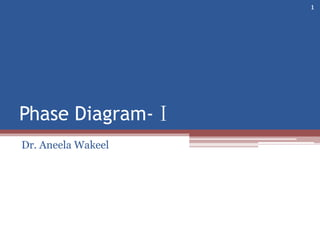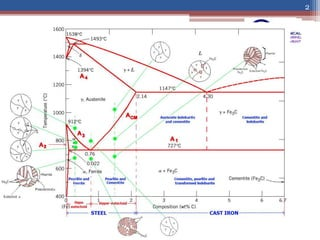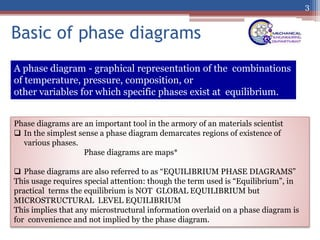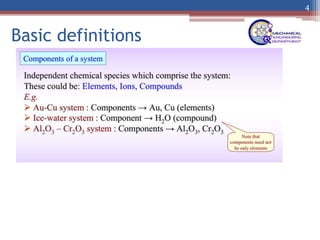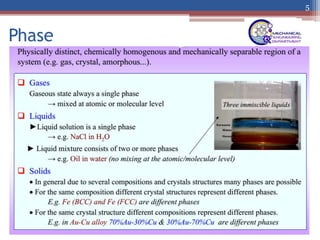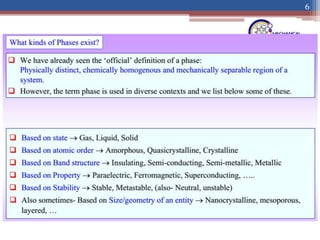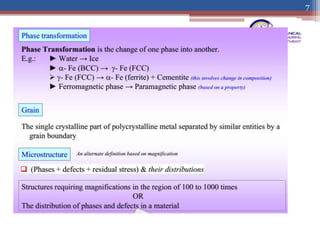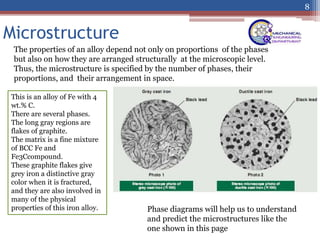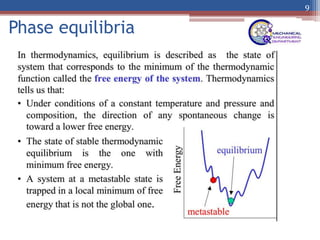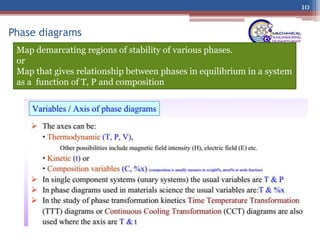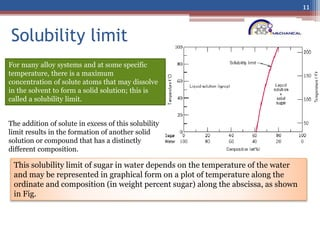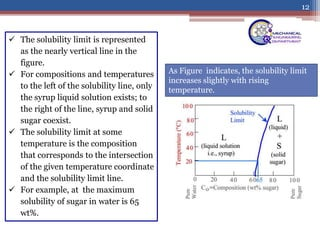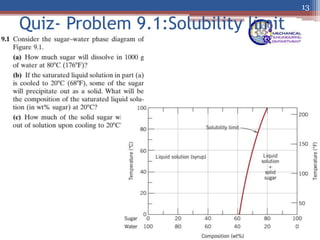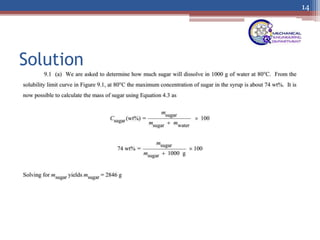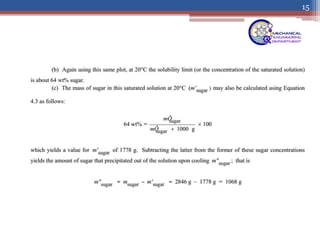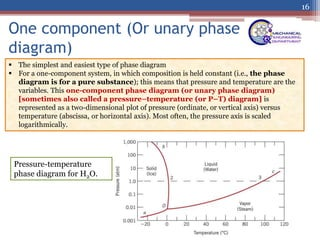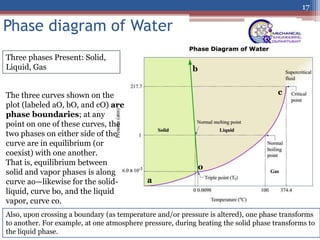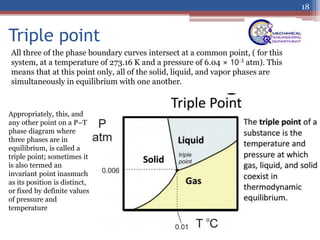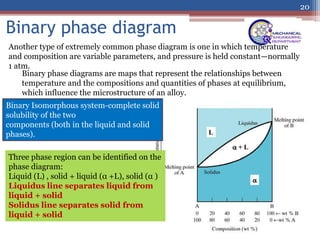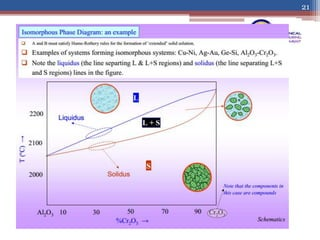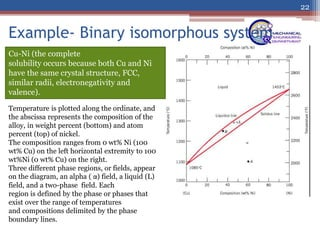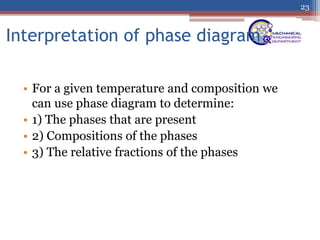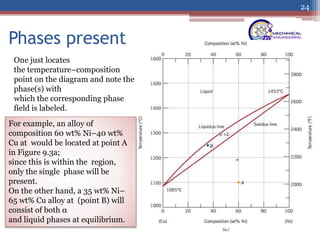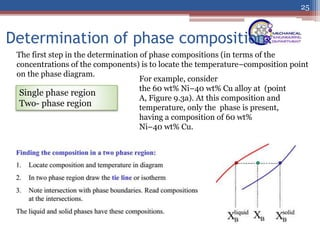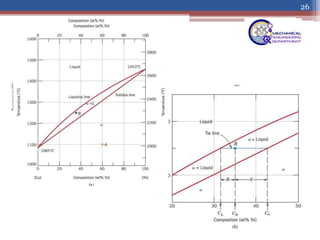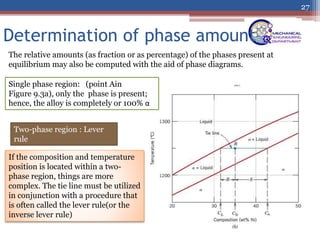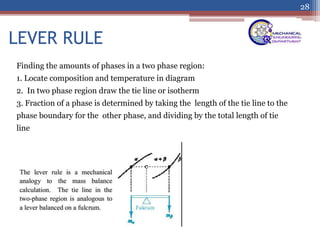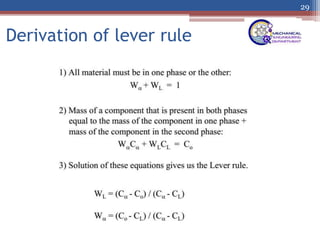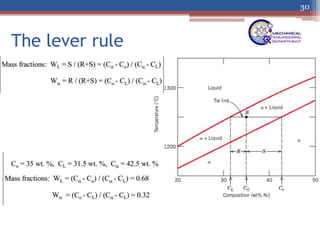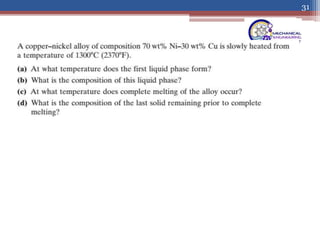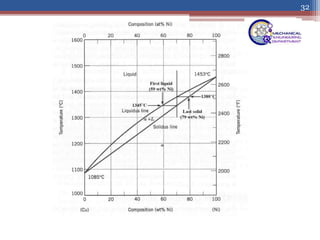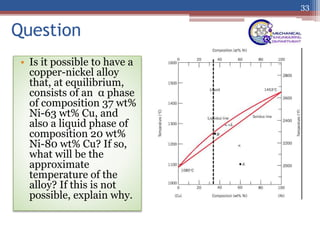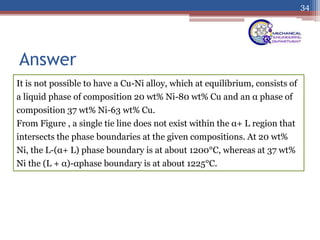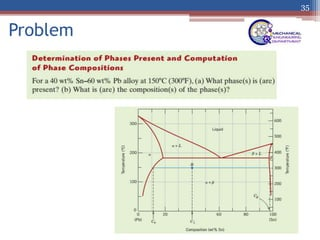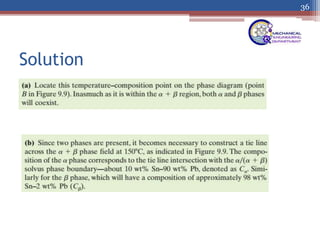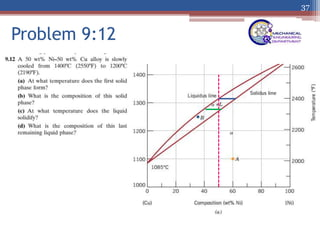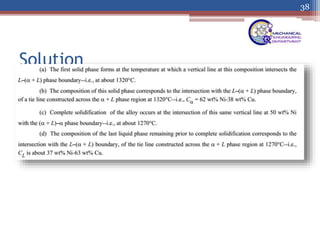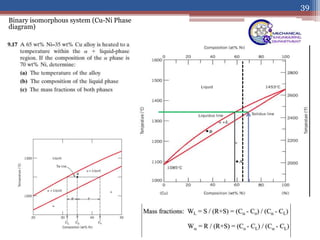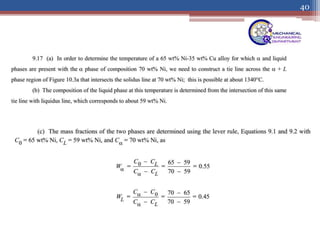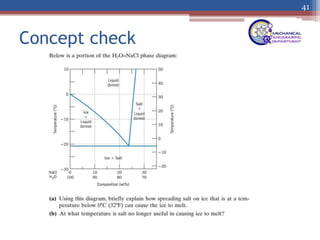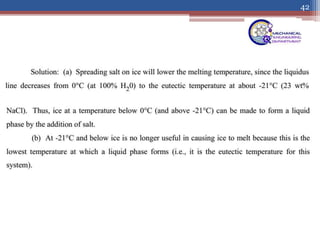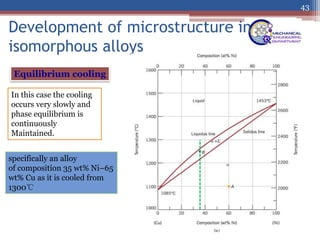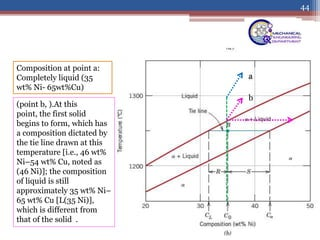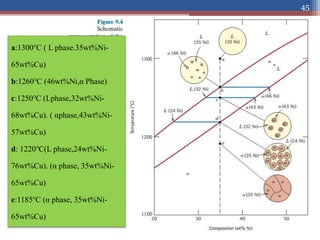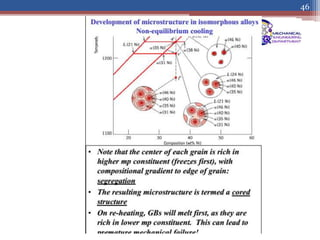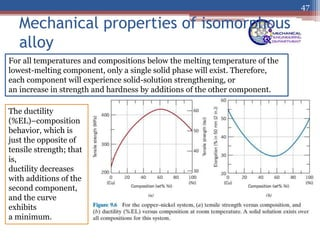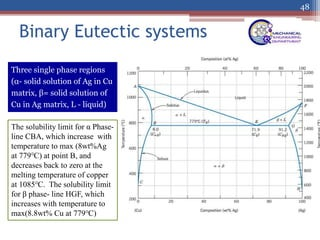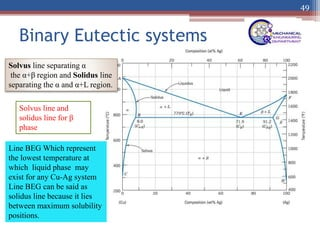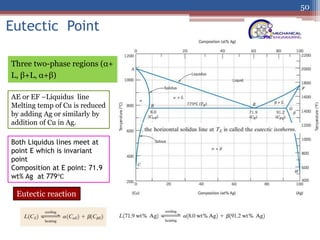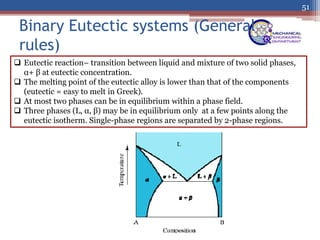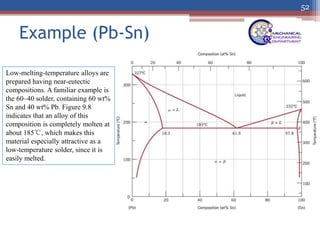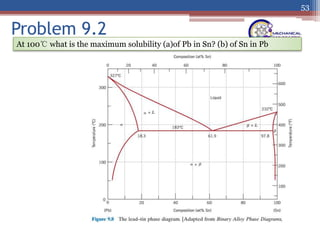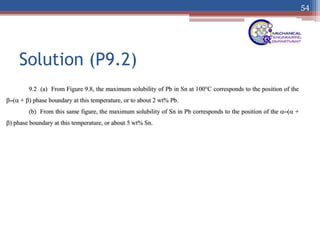1 de 54

### PHASE DAIGRAM 1.pptx

• 2. 2
• 3. Basic of phase diagrams 3 Phase diagrams are an important tool in the armory of an materials scientist  In the simplest sense a phase diagram demarcates regions of existence of various phases. Phase diagrams are maps*  Phase diagrams are also referred to as “EQUILIBRIUM PHASE DIAGRAMS” This usage requires special attention: though the term used is “Equilibrium”, in practical terms the equilibrium is NOT GLOBAL EQUILIBRIUM but MICROSTRUCTURAL LEVEL EQUILIBRIUM This implies that any microstructural information overlaid on a phase diagram is for convenience and not implied by the phase diagram. A phase diagram - graphical representation of the combinations of temperature, pressure, composition, or other variables for which specific phases exist at equilibrium.
• 6. 6
• 7. 7
• 8. Microstructure 8 The properties of an alloy depend not only on proportions of the phases but also on how they are arranged structurally at the microscopic level. Thus, the microstructure is specified by the number of phases, their proportions, and their arrangement in space. This is an alloy of Fe with 4 wt.% C. There are several phases. The long gray regions are flakes of graphite. The matrix is a fine mixture of BCC Fe and Fe3Ccompound. These graphite flakes give grey iron a distinctive gray color when it is fractured, and they are also involved in many of the physical properties of this iron alloy. Phase diagrams will help us to understand and predict the microstructures like the one shown in this page
• 10. Phase diagrams 10 Map demarcating regions of stability of various phases. or Map that gives relationship between phases in equilibrium in a system as a function of T, P and composition
• 11. Solubility limit 11 For many alloy systems and at some specific temperature, there is a maximum concentration of solute atoms that may dissolve in the solvent to form a solid solution; this is called a solubility limit. The addition of solute in excess of this solubility limit results in the formation of another solid solution or compound that has a distinctly different composition. This solubility limit of sugar in water depends on the temperature of the water and may be represented in graphical form on a plot of temperature along the ordinate and composition (in weight percent sugar) along the abscissa, as shown in Fig.
• 12. 12  The solubility limit is represented as the nearly vertical line in the figure.  For compositions and temperatures to the left of the solubility line, only the syrup liquid solution exists; to the right of the line, syrup and solid sugar coexist.  The solubility limit at some temperature is the composition that corresponds to the intersection of the given temperature coordinate and the solubility limit line.  For example, at the maximum solubility of sugar in water is 65 wt%. As Figure indicates, the solubility limit increases slightly with rising temperature.
• 15. 15
• 16. One component (Or unary phase diagram) 16  The simplest and easiest type of phase diagram  For a one-component system, in which composition is held constant (i.e., the phase diagram is for a pure substance); this means that pressure and temperature are the variables. This one-component phase diagram (or unary phase diagram) [sometimes also called a pressure–temperature (or P–T) diagram] is represented as a two-dimensional plot of pressure (ordinate, or vertical axis) versus temperature (abscissa, or horizontal axis). Most often, the pressure axis is scaled logarithmically. Pressure-temperature phase diagram for H2O.
• 17. Phase diagram of Water 17 Three phases Present: Solid, Liquid, Gas The three curves shown on the plot (labeled aO, bO, and cO) are phase boundaries; at any point on one of these curves, the two phases on either side of the curve are in equilibrium (or coexist) with one another. That is, equilibrium between solid and vapor phases is along curve ao—likewise for the solid- liquid, curve bo, and the liquid vapor, curve co. o a b c Also, upon crossing a boundary (as temperature and/or pressure is altered), one phase transforms to another. For example, at one atmosphere pressure, during heating the solid phase transforms to the liquid phase.
• 18. Triple point 18 All three of the phase boundary curves intersect at a common point, ( for this system, at a temperature of 273.16 K and a pressure of 6.04 × 10-3 atm). This means that at this point only, all of the solid, liquid, and vapor phases are simultaneously in equilibrium with one another. Appropriately, this, and any other point on a P–T phase diagram where three phases are in equilibrium, is called a triple point; sometimes it is also termed an invariant point inasmuch as its position is distinct, or fixed by definite values of pressure and temperature
• 20. Binary phase diagram 20 Binary phase diagrams are maps that represent the relationships between temperature and the compositions and quantities of phases at equilibrium, which influence the microstructure of an alloy. Another type of extremely common phase diagram is one in which temperature and composition are variable parameters, and pressure is held constant—normally 1 atm. Binary Isomorphous system-complete solid solubility of the two components (both in the liquid and solid phases). Three phase region can be identified on the phase diagram: Liquid (L) , solid + liquid (α +L), solid (α ) Liquidus line separates liquid from liquid + solid Solidus line separates solid from liquid + solid
• 21. 21
• 22. Example- Binary isomorphous system 22 Cu-Ni (the complete solubility occurs because both Cu and Ni have the same crystal structure, FCC, similar radii, electronegativity and valence). Temperature is plotted along the ordinate, and the abscissa represents the composition of the alloy, in weight percent (bottom) and atom percent (top) of nickel. The composition ranges from 0 wt% Ni (100 wt% Cu) on the left horizontal extremity to 100 wt%Ni (0 wt% Cu) on the right. Three different phase regions, or fields, appear on the diagram, an alpha ( α) field, a liquid (L) field, and a two-phase field. Each region is defined by the phase or phases that exist over the range of temperatures and compositions delimited by the phase boundary lines.
• 23. Interpretation of phase diagram • For a given temperature and composition we can use phase diagram to determine: • 1) The phases that are present • 2) Compositions of the phases • 3) The relative fractions of the phases 23
• 24. Phases present 24 One just locates the temperature–composition point on the diagram and note the phase(s) with which the corresponding phase field is labeled. For example, an alloy of composition 60 wt% Ni–40 wt% Cu at would be located at point A in Figure 9.3a; since this is within the region, only the single phase will be present. On the other hand, a 35 wt% Ni– 65 wt% Cu alloy at (point B) will consist of both α and liquid phases at equilibrium.
• 25. Determination of phase composition 25 The first step in the determination of phase compositions (in terms of the concentrations of the components) is to locate the temperature–composition point on the phase diagram. Single phase region Two- phase region For example, consider the 60 wt% Ni–40 wt% Cu alloy at (point A, Figure 9.3a). At this composition and temperature, only the phase is present, having a composition of 60 wt% Ni–40 wt% Cu.
• 26. 26
• 27. Determination of phase amount 27 The relative amounts (as fraction or as percentage) of the phases present at equilibrium may also be computed with the aid of phase diagrams. Two-phase region : Lever rule If the composition and temperature position is located within a two- phase region, things are more complex. The tie line must be utilized in conjunction with a procedure that is often called the lever rule(or the inverse lever rule) Single phase region: (point Ain Figure 9.3a), only the phase is present; hence, the alloy is completely or 100% α
• 28. LEVER RULE 28 Finding the amounts of phases in a two phase region: 1. Locate composition and temperature in diagram 2. In two phase region draw the tie line or isotherm 3. Fraction of a phase is determined by taking the length of the tie line to the phase boundary for the other phase, and dividing by the total length of tie line
• 31. 31
• 32. 32
• 33. Question • Is it possible to have a copper-nickel alloy that, at equilibrium, consists of an α phase of composition 37 wt% Ni-63 wt% Cu, and also a liquid phase of composition 20 wt% Ni-80 wt% Cu? If so, what will be the approximate temperature of the alloy? If this is not possible, explain why. 33
• 34. Answer 34 It is not possible to have a Cu-Ni alloy, which at equilibrium, consists of a liquid phase of composition 20 wt% Ni-80 wt% Cu and an α phase of composition 37 wt% Ni-63 wt% Cu. From Figure , a single tie line does not exist within the α+ L region that intersects the phase boundaries at the given compositions. At 20 wt% Ni, the L-(α+ L) phase boundary is at about 1200°C, whereas at 37 wt% Ni the (L + α)-αphase boundary is at about 1225°C.
• 39. Binary isomorphous system (Cu-Ni Phase diagram) 39
• 42. 42
• 43. Development of microstructure in isomorphous alloys 43 Equilibrium cooling In this case the cooling occurs very slowly and phase equilibrium is continuously Maintained. specifically an alloy of composition 35 wt% Ni–65 wt% Cu as it is cooled from 1300℃
• 44. 44 a Composition at point a: Completely liquid (35 wt% Ni- 65wt%Cu) b (point b, ).At this point, the first solid begins to form, which has a composition dictated by the tie line drawn at this temperature [i.e., 46 wt% Ni–54 wt% Cu, noted as (46 Ni)]; the composition of liquid is still approximately 35 wt% Ni– 65 wt% Cu [L(35 Ni)], which is different from that of the solid .
• 45. 45 a:1300℃ ( L phase.35wt%Ni- 65wt%Cu) b:1260℃ (46wt%Ni,α Phase) c:1250℃ (Lphase,32wt%Ni- 68wt%Cu). ( αphase,43wt%Ni- 57wt%Cu) d: 1220℃(L phase,24wt%Ni- 76wt%Cu). (α phase, 35wt%Ni- 65wt%Cu) e:1185℃ (α phase, 35wt%Ni- 65wt%Cu)
• 46. 46
• 47. Mechanical properties of isomorphous alloy 47 For all temperatures and compositions below the melting temperature of the lowest-melting component, only a single solid phase will exist. Therefore, each component will experience solid-solution strengthening, or an increase in strength and hardness by additions of the other component. The ductility (%EL)–composition behavior, which is just the opposite of tensile strength; that is, ductility decreases with additions of the second component, and the curve exhibits a minimum.
• 48. Binary Eutectic systems 48 Three single phase regions (α- solid solution of Ag in Cu matrix, β= solid solution of Cu in Ag matrix, L - liquid) The solubility limit for α Phase- line CBA, which increase with temperature to max (8wt%Ag at 779℃) at point B, and decreases back to zero at the melting temperature of copper at 1085℃. The solubility limit for β phase- line HGF, which increases with temperature to max(8.8wt% Cu at 779℃)
• 49. Binary Eutectic systems 49 Solvus line separating α the α+β region and Solidus line separating the α and α+L region. Solvus line and solidus line for β phase Line BEG Which represent the lowest temperature at which liquid phase may exist for any Cu-Ag system Line BEG can be said as solidus line because it lies between maximum solubility positions.
• 50. Eutectic Point 50 Three two-phase regions (α+ L, β+L, α+β) AE or EF –Liquidus line Melting temp of Cu is reduced by adding Ag or similarly by addition of Cu in Ag. Both Liquidus lines meet at point E which is invariant point Composition at E point: 71.9 wt% Ag at 779℃ Eutectic reaction
• 51. Binary Eutectic systems (General rules) 51  Eutectic reaction– transition between liquid and mixture of two solid phases, α+ β at eutectic concentration.  The melting point of the eutectic alloy is lower than that of the components (eutectic = easy to melt in Greek).  At most two phases can be in equilibrium within a phase field.  Three phases (L, α, β) may be in equilibrium only at a few points along the eutectic isotherm. Single-phase regions are separated by 2-phase regions.
• 52. Example (Pb-Sn) 52 Low-melting-temperature alloys are prepared having near-eutectic compositions. A familiar example is the 60–40 solder, containing 60 wt% Sn and 40 wt% Pb. Figure 9.8 indicates that an alloy of this composition is completely molten at about 185℃, which makes this material especially attractive as a low-temperature solder, since it is easily melted.
• 53. Problem 9.2 53 At 100℃ what is the maximum solubility (a)of Pb in Sn? (b) of Sn in Pb

### Notas del editor

1. Above the critical pressure and temperature, there is no distinction between the liquid phase and the gas phase. Basically, they merge into one phase that is called the super critical fluid phase (SCF).